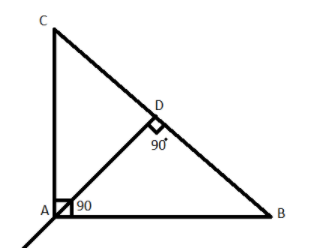A right angle triangle ABC is right angled at A, then the orthocenter of triangle ABC is at:$\left( a \right)$ C$\left( b \right)$ A$\left( c \right)$ B$\left( d \right)$ None of theseVerified
141.9k+ views
Hint: In this particular question use the concept that the orthocenter of any triangle is the intersection of the altitudes drawn from the vertices of the triangle on the opposite sides of the vertices so use these concepts to reach the solution of the question.